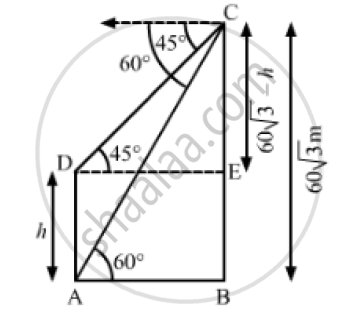# The Angles of Depression of the Top and Bottom of a Tower as Seen from the Top of a 60 Sqrt(3) M High Cliff Are 45° and 60° Respectively. Find the Height of the Tower. - Mathematics

The angles of depression of the top and bottom of a tower as seen from the top of a 60  sqrt(3) m high cliff are 45° and 60° respectively. Find the height of the tower.

#### SolutionLet AD be the tower and BC be the cliff.
We have,
BC = 60 sqrt(3) , ∠ CDE = 45° and ∠BAC = 60°
⇒ BE = AD = h
⇒ CE =  BC - BE= 60 sqrt(3)  -  h
In  ΔCDE,

 tan 45° = (CE)/(DE)

⇒ 1 = (60 sqrt(3) -h)/(DE)

⇒ DE = 60 sqrt(3) - h

⇒ AB = DE = 60 sqrt(3) - h                      ............(1)

Now, in ΔABC

tan 60° = (BC)/(AB)

⇒ sqrt(3)= (60 sqrt(3) )/ (60 sqrt(3) -h)                 [ Using (1)]

⇒ 180 - h sqrt(3) = 60 sqrt(3)

⇒ h sqrt(3) = 180- 60 sqrt(3)

⇒ h = (108 -60sqrt(3) )/sqrt(3) xx sqrt(3)/sqrt(3)

⇒  h = ( 180 sqrt(3)-180) /3

⇒ h = (180 sqrt(3)-1) /3

∴ h = 60 ( sqrt(3)-1)

= 60 (1.732 -1)

= 60 (0.7.32)

Also, h = 43.92m

So, the height of the tower is 43. 92 m.

Concept: Heights and Distances
Is there an error in this question or solution?
Chapter 14: Height and Distance - Exercises

#### APPEARS IN

RS Aggarwal Secondary School Class 10 Maths
Chapter 14 Height and Distance
Exercises | Q 22

Share